# Heap Maps 101

##### 14minutes

Good news. I created another tutorial on heap maps that will live on the web. And this one includes a bunch of colorful diagrams. So a heap map, also known as a heap, is a set of values stored in a specific order that allows efficient access or modification. Heap maps are usually represented in a tree structure and only two sorting directions the tree's values can be stored. There are min heaps where the smallest number is represented at the top or a max heap where the largest number is represented at the top.## Why use a heap map

To decide if a heap map is the right thing to use we have to first look at the problem we are trying to solve. If you want to find the smallest or largest value quickly than a heap map is the answer. This is because we are always sorting the heap when elements are entering or leaving. One of the biggest benefits of this implementation is not having to look at every element when sorting. In tree structures, after looking at the first row of children and determining which branch to follow, we are eliminating having to look at least half of the tree. In small heap maps the benefits may not be apparent, but with larger heap maps the benefits are plenty.

The most common implementation of heaps are the binary kind. This is where a parent element can only have up to two children. And we can track the parent's children using a solution like this.

``````class Node {
constructor(val, left, right) {
this.value = val;
this.left = null;
this.right = null;
}
}``````

The reason why binary heap maps are so common is because instead of worrying about linking and keeping track of elements, we can take advantage of using an array to store and sort values. We will always be able to find a parent's left child by using this fail proof -- super complicated -- formula of `index * 2` and the right child by adding one to the left child. As an alternative we can find a child's parent by doing another fool proof -- even more complicated -- formula of `index / 2`.

Examples of Heap Maps

We first start by adding the new element to the end of the array.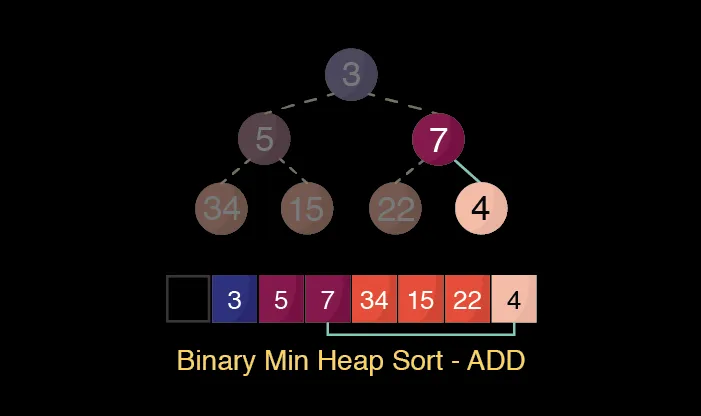``this.heap.push(element);``

We then need to compare this new element to its parent to see if it smaller or larger. In this case 2 is smaller then 7. We can swap the two elements.``````if (parent <= element) { break; } // false
else {
this.heap[parentN] = element;
this.heap[n] = parent;
}``````

The first loop is over so we start the comparisons again

We then need to compare the new element to its parent to see if it smaller or larger. In this case 4 is NOT smaller than 3. Therefore it can stay at its current index.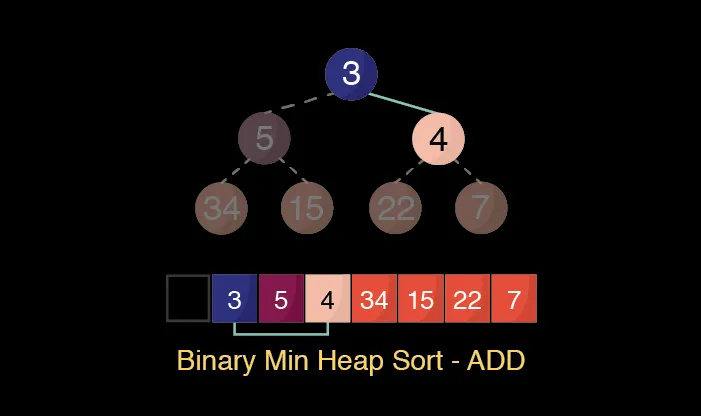``````if (parent <= element) { break; } // true
else {
this.heap[parentN] = element;
this.heap[n] = parent;
}``````

YAY the heap is in order!!!!## POP

When an element is removed it creates a hole in the array. We need to fill that hole with the last element in the array.

We would then need to shuffle the element down into the correct balanced position. By doing so we are comparing the new element with its children and swapping array indexes when needed.

This can be done on logarithmic time. Θ(log n)

Let's begin.We need to remove the first index by replacing it with the last index in the array.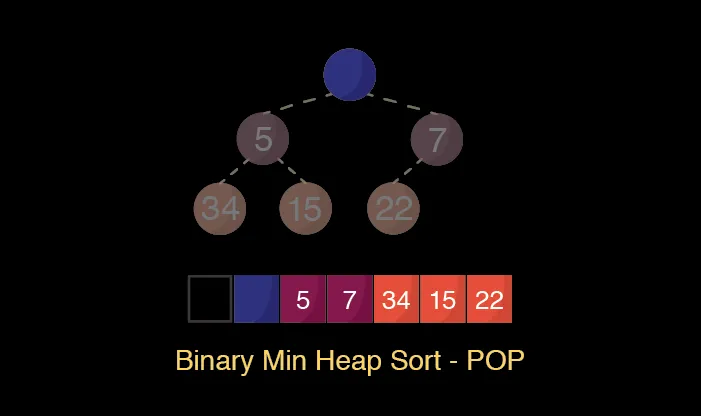``const peek = this.heap;````const end = this.heap.pop(); this.heap = end;``

Next, check the left child against the parent to see if it is smaller. In this case 5 is smaller than 22. Now let's save the index number of the left child which is 2.

!["Binary Min Heap Pop Step 3"](/img/heap-maps-101/pop-min-heap-4-wide.png "Binary Min Heap Pop Step 3")

``````var leftScore = this.compare(parent, leftChild);
if (leftScore > 0) { // true
swapN = leftN;
}``````

We will do the same comparison to the right child. In this case 7 is smaller than 22.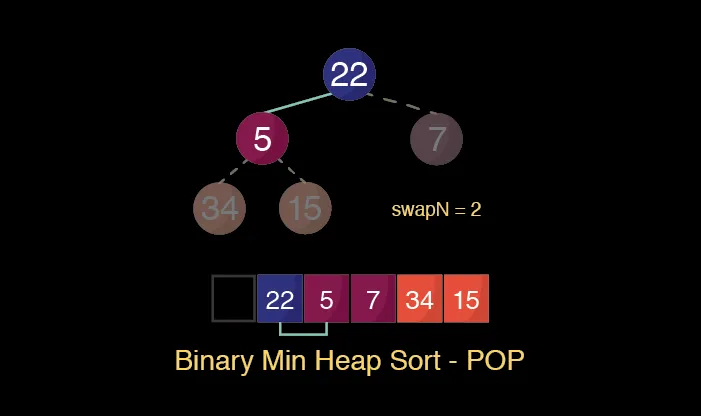``const rightScore = this.compare(parent, rightChild);``

But before we can save the right child's index we need to compare it to the left child. 5 is smaller than 7 so we actually don't need to save the the right child's index.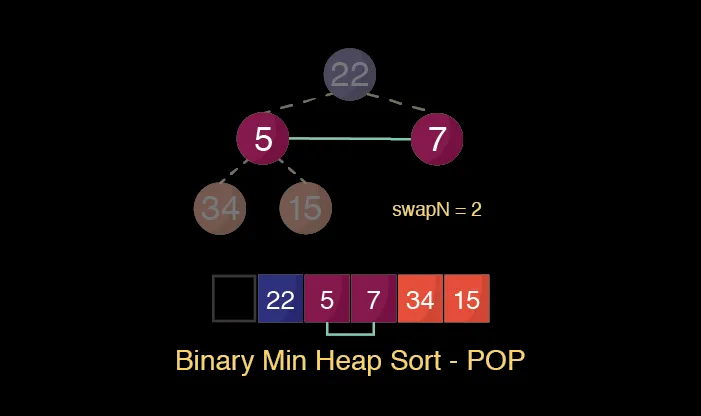``````if (rightScore > leftScore) { // false
swapN = rightN;
}``````

Now we swap the indexes between the parent and the left child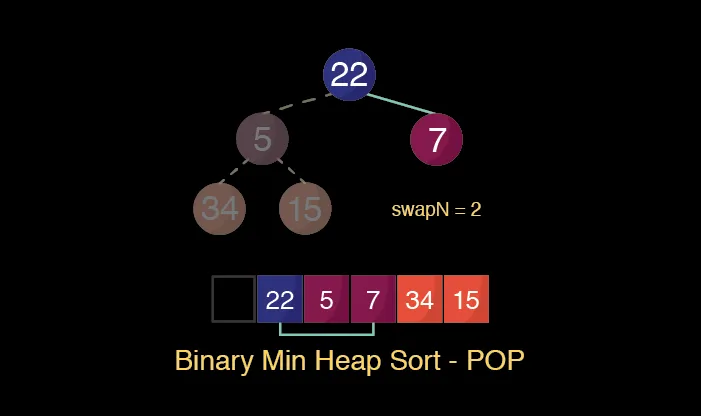``````// swapN = leftN
this.heap[n] = this.heap[swapN];
this.heap[swapN] = parent;
n = swapN;``````

The first loop is over so we start the comparisons again

***

We need to check the left child against the parent to see if it is smaller. In this case 34 is NOT smaller than 22. So we do NOT save the index number of the left child.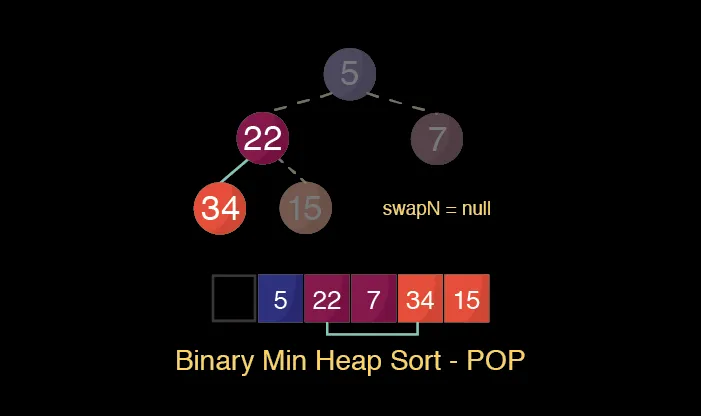``````var leftScore = this.compare(parent, leftChild);
if (leftScore > 0) { // false
swapN = leftN;
}``````

Next, we comparison the right child to its parent. In this case 15 is smaller than 22. Since we haven't saved a "swap" index and we know the child is smaller than its parent, we can save the index number of the right child which is 5.``````const rightScore = this.compare(parent, rightChild);
if ((swapN == null && rightScore > 0)) { // true
swapN = rightN;
}``````

Now we swap the indexes between the parent and child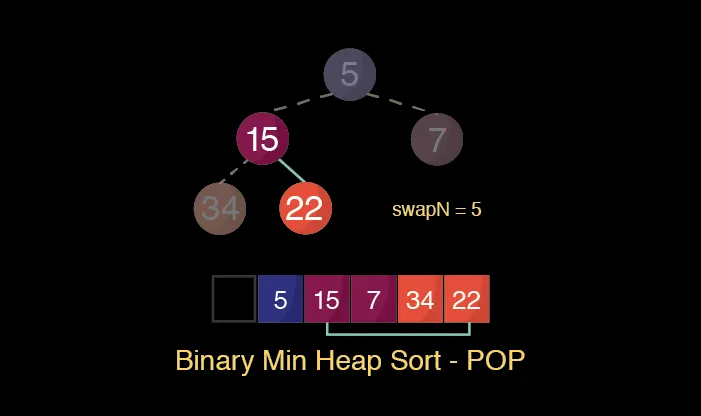``````// swapN = rightN
this.heap[n] = this.heap[swapN];
this.heap[swapN] = parent;
n = swapN;``````

YAY the heap is in order!!!!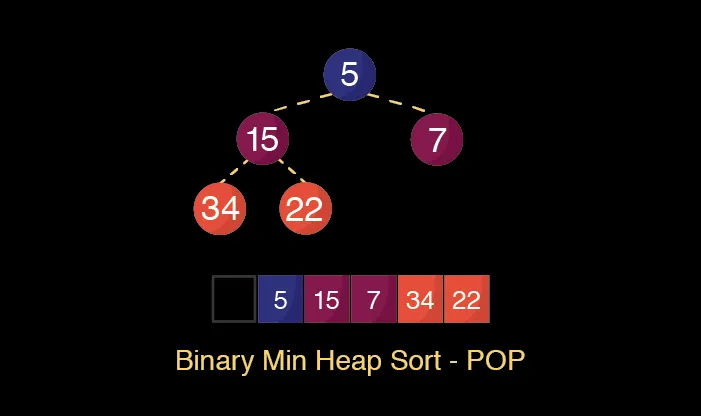When learning how to implement an heap map in JavaScript I followed Eloquent JavaScript heap map implementation. It has a score function, which I didn't found very useful. So I created a `compare` function that determines if the parent is larger by returning a positive number.

You might have also noticed I left the array index 0 as null. By no means do you need to do the same. When learning how to implement an heap maps most tutorials I followed were not in JavaScript and left the the first index 0 as well. And I wanted to have a good comparison when looking at other tutorials.

Now the part we have all been waiting for. The full code!!!

``````class BinaryHeap {
constructor(compare) {
this.heap = [null];
this.compare = compare || this.compareFunction;
}
/**
* Get the length of the heap
*
* return number
*/
size() {
return this.heap.length;
}

/**
* Determine if the parent is larger then its child.
* Returning a positive number means parent is larger
* @param {number} parent
* @param {number} child
*
* return number
*/
compareFunction(parent, child) {
return parent - child;
}

/**
* Get the peek element fill the in the hole with the last element in array
* Then sort the replaced element.
*
* return number
*/
pop() {
const peek = this.heap; // 1
const end = this.heap.pop(); // 9
if (this.size() > 1) {
this.heap = end;
this.sinkDown(1);
}
return peek;
}

/**
* Add new elements to the end of the array then sort
*
* @param {number} element
*
* return null
*/
this.heap.push(element);
this.bubbleUp(this.size() - 1);
}

/**
* Sorting the current element by comparing it parent to see
* if it is smaller
*
* @param {number} n the index number of the current element
*/
bubbleUp(n) {
const element = this.heap[n];
// When at 1, we are at the peek.
while (n > 1) {
// Compute the parent element's index, and fetch it.
const parentN = Math.floor((n + 1) / 2);
const parent = this.heap[parentN];
// if we get a positive number, then the parent is larger
// and we can leave it in its current index
if (this.compare(parent, element) < 0) break;

// Otherwise swap the parent and child
this.heap[parentN] = element;
this.heap[n] = parent;
n = parentN;
}
}

sinkDown(n) {
const length = this.size();
const parent = this.heap[n];

while (true) { // we break out the loop when we can no longer swap elements
// Get child elements indexes.
const leftN = n * 2;
const rightN = leftN + 1;
// This is used to store the new position of the element
let swapN = null;
// If the first child exists (is inside the array)...
if (leftN < length) {
const leftChild = this.heap[leftN];
// positive result means the parent is larger
var leftScore = this.compare(parent, leftChild);
if (leftScore > 0) swapN = leftN;
}
// Do the same checks for the other child.
if (rightN < length) {
const rightChild = this.heap[rightN];
const rightScore = this.compare(parent, rightChild);
// check if swap is null and parent is bigger
// Otherwise if the rightScore is bigger it means its the smaller child
// we need to set the swap index to the smaller child index
if ((swapN == null && rightScore > 0) || rightScore > leftScore) {
swapN = rightN;
}
}

// No need to swap further, we are done.
if (swapN == null) break;

// Otherwise, swap and continue the loop.
this.heap[n] = this.heap[swapN];
this.heap[swapN] = parent;
n = swapN;
}
}
}
```

Try it our ourself.
```javascript
const heap = new BinaryHeap();
[34, 15, 22, 7, 5, 3].forEach(ele => {